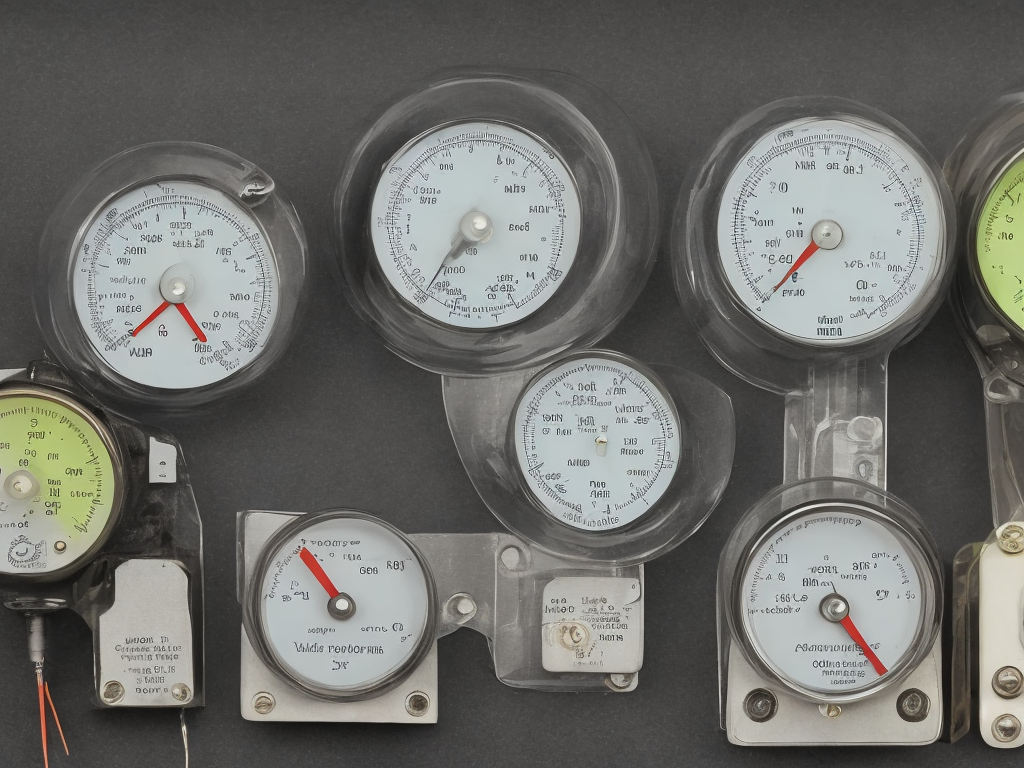# Difference Between Ammeter And Voltmeter

tl;dr
An ammeter measures current (in amperes) and a voltmeter measures voltage (in volts).An ammeter measures current, while a voltmeter measures voltage. An ammeter is connected in series with the circuit, while a voltmeter is connected in parallel.

An ammeter measures the amount of current flowing through a circuit, and is measured in amperes (A). This is useful to measure how much power is being used by a particular circuit, and to detect any possible short circuits.

A voltmeter measures the voltage of a circuit, and is measured in volts (V). This is useful to measure the energy of a circuit, and to detect any possible open circuits.

In summary, an ammeter measures current, while a voltmeter measures voltage. Both are important tools for testing and troubleshooting electrical circuits.